# Voting model totally unrelated to anything in real life

Suppose there is a town that is totally not a fictionalized version of a real life scenario. The town is considering building a dog park and funding the construction of the dog park through a ballot measure that would raise taxes by [insert uncontroversial number here]. If at least 25% of the town’s population votes for the ballot measure and at least $\frac{2}{3}$ of the voters approve the measure, it passes.

1. There are 17,000 people in the town
2. There are 300 dog owners who will be directly impacted and who are assumed to strongly support the ballot measure.
3. The ballet measure would also provide some funding for improvement of the towns other parks.
4. Approximately 40% of the town residents routinely use the parks. Assume these residents are generally inclined to support the measure on average but the intensity of their support is uncertain and variable.

# Assurance 1

I talk a lot in this post about probabilities and probability models but rest assured I’m not actually doing anything complicated. What I have here is actually simple possibly to the point of being trivial or sloppy.

Related to Assurance 1, there is a lot of pretend randomness in these models I cooked up. I wanted to set this up using a sort of probability framework so I could couch it as ‘simulating hypothetical elections’ under different circumstances. I’ll freely admit that what I have here is kind of overkill since, with the parameters I deem reasonable, results are pretty much driven by average voting propensities of whatever the largest group is.

I might spend a little more time thinking about how to either simplify this presentation or make it complicated enough that some volatility in simulated results is introduced.

Voter turnout is an important hurdle in the set-up I have proposed. The Ballot Measure I described here can only pass if it gets two-thirds of the vote and if at least one-quarter of the town’s population shows up to vote. I built this criteria into the models below but it actually turns out to be rather unimportant in my simulations. This is mostly because I generally choose values for the ad-hoc parameters that produce total voter-turnout figures around 40% (since this is what we observe most often in real life). Like I said, the voter turnout criteria is baked-in to my models but I don’t talk it much since it turns out not to be too important.

Here is my, how to interpret this pitch: I didn’t set this up because I thought I knew exactly how to estimate or forecast the probability of a ballot measure passing or failing. I set it up as a way to systematically examine some of the effects of different assumptions about:

1. the underlying make up of the town population (how many do we think will definitely vote for the dog park, how many do we think will definitely vote against it, how many are undecided and could possibly be convinced to vote in favor?)
2. the probabilities associated with each voter type (for residents who are likely to vote in favor is it like 9 out of 10 these will vote yes? or is it more like 6 out of 10? for residents that are undecided, do we think 5 out of 10 of these will vote for it? what if we were able to get 6 or 7 out 10 of the undecided voters).

If you think my assuptions don’t paint an accurate picture of the town population viz-a-viz their feelings toward the proposed dog-park, I’ve posted my code here so you can just change the parameters you don’t like and run it your way. Or just tell me what you think the parameter values should be and I’ll run some new simulations.

## A Basic Referendum Model

The rules of the ballet measure stipulate that, in order for the dog-park referendum to pass, the number of ‘yes’ votes needs to meet or exceed 66% of the voters AND at least 25% of the town population needs to vote.

We start by observing that the probability that any individual resident votes ‘yes’ on the referendum is the product of the probability that the resident votes by the probability that the resident votes ‘yes’ conditional on voting:

Here:

is the probability that resident $i$ votes “yes” if resident $i$ shows up to vote.

$p_{i}(yes)$ probability that resident $i$ votes yes.

$p_{i}(vote)$ is the probability that resident $i$ shows up to vote.

This problem can be framed like a coin toss experiment. Each resident can be thought of as two flips of the coin: the first flip determines whether the resident votes or not and the second flip decided whether the resident votes yes. In this case the probability of voting and probability of voting yes conditional on voting define the odds of these two occurances.

The expected total number of successes in $N$ independent trials if each trial has a $p$ probability of success is, $Np$. In this case, in order to get a yes vote, we actually need two successes (we need vote=yes and vote yes = yes). This is expressed similarly for the binominal distribution…assuming for the moment that all residents have the same probability of voting and the same probability of voting yes:

where $N$ is the total resident population.

As noted above, in order to pass the referendum two conditions need to be met:

1. $\frac{\sum_{i}yes_{i}}{\sum_{i}vote_{i}}>0.66$
2. $\frac{\sum_{i}vote_{i}}{17000}>0.25$

## Data and Fixed Parameters

In most of the simulations I assume that voter turnout will hoover somewhere in the 40% neighborhood and generally choose other parameter values that are consistent with 40% voter turn-out.

## Parameters to vary

• $pvote(type)$ this is the probability that a resident of type type shows up to vote
• $pyes(type)$ this is the probability that a resident of type type votes ‘yes’ conditional on that person voting
• $pop(type)$ this is the town-wide total population for each type

## Assumptions

If all residents have the same probability of voting ‘yes’ then the problem is trivial and not really worth discussing further.

I want to examine some slightly more realistic scenarios:

## A 3 type model

To start, we assume that there are three types of residents:

• those who are highly likely to vote and highly likely to vote against the dog park
• those who are highly likely to vote and highly likely to vote in favor of the dog park
• those who are ambivalent: their liklihood of voting is variable and the probability they vote in favor of the dog park (conditional on voting at all) is also variable

### A simple example

Let’s begin by labeling the three groups A, B, C such that:

• A is the group with a high probability of voting and a high probability of voting ‘yes’ on the dog park
• B is the group with a high probability of voting and a high probability of voting ‘no’ on the dog park
• C is the group of residents not super motivated to vote and have a highly variable probability of voting ‘yes’ if they do show up to vote.

To make things a little more concrete assume that:

• Group A represents 3% of the town (roughly 500 residents) and this group has a 95% chance of showing up to vote and only a 98% chance of voting ‘yes’. We can think of this group as the dog owners plus close friends
• Group B represents 3% of the town population (roughly 500 residents), has a 95% chance of showing up to vote and a 2% of voting ‘yes’.
• Group C is the remainder (94% of the town ~16,000), has probability of $pC.vote$ of showing up to vote and a 50% chance of voting ‘yes’ if they do show up

Assuming that Groups A and B have a very strong probability (on average) of showing up to vote, a vote probability for Group C that would be consistent with 40% total voter turn-out would be 0.4.

If $pC.vote = 0.4$ and 5 out every 10 members of Group C on average vote ‘yes’, how would the dog park referendum turn out?

The expected number of votes from groups A, B, and C are calculated as:

The expected number of ‘yes’ votes is:

$E(yes_{A}) = E(vote_{A})*0.98$ probability type A votes ‘yes’ given that they vote

$E(yes_{B}) = E(vote_{B})*0.02$ probability type B votes ‘yes’ given that they vote

$E(yes_{C}) = E(vote_{C})*0.5$ probability type C votes ‘yes’ given that they vote

With these parameters the percent of yes votes is:

$\frac{E(yes_{A})+E(yes_{B})+E(yes_{C})}{E(vote_{A})+E(vote_{B})+E(vote_{C})} = 0.5$

And total expected voter turnout is:

$\frac{E(vote_{A})+E(vote_{B})+E(vote_{C})}{17000}=0.433$

In this example, because the high probability yes voters are equal to the high probability no voters in number and in likelihood of voting, they essentially cancel each other out. This means the final result is driven by the propensity of Group C to vote yes.

The final count of yes votes as a proportion of total votes is linear in the parameter

as is illustrated below. Note that in the plot below I make a few minor modifications:

1. I assume that Type A voters are 4% of the town population
2. I assume that Type B voters are only 2% of the town population

#===============================================================================
#===============================================================================
#===============================================================================
simple.fn <- function(ratio,pA,pB,pC,popA,pA.vote,pB.vote,pC.vote){

popB <- ratio*popA
popC <- 17000-(popA+popB)

turnoutA <- popA*pA.vote
turnoutB <- popB*pB.vote
turnoutC <- popC*pC.vote

yesA <- turnoutA*pA
yesB <- turnoutB*pB
yesC <- turnoutC*pC

yespct <- (yesA+yesB+yesC)/(turnoutA+turnoutB+turnoutC)
turnout <- (turnoutA + turnoutB + turnoutC)/17000

win <- 0
if(turnout>=0.25 & yespct>=0.666){win=1}
return(data.frame(pA=pA,pB=pB,pC=pC,
pA.vote=pA.vote,pB.vote=pB.vote,pC.vote=pC.vote,
popA=popA,popB=popB,popC=popC,
yespct=yespct,turnout=turnout,win=win))
}

#apply this function over different values of gen pop pct yes
scen1 <- data.frame(rbindlist(lapply(c(seq(from=0.5,to=0.7,by=0.025)),simple.fn,pA=0.98,pB=0.02,
pA.vote=0.95,pB.vote=0.95,pC.vote=.39,
popA=0.04*17000,ratio=0.5)))

ggplot(scen1,aes(x=pC,y=yespct)) + geom_line() + geom_point() +
geom_hline(yintercept=0.66,color='red') + theme_bw() +
xlab('Type C p(yes)') + ylab('Total YES percent')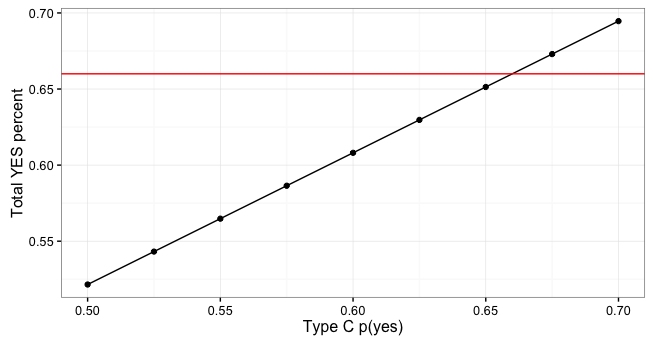The graph above is trivial but not uninformative. One way to look at is this:

Even if you believe that

1. there are twice as many people strongly in favor of the dog park as there are people strongly opposed to the dog park, AND
2. the majority of the 17,000 residents are neither strongly opposed nor strongly in favor, Then
3. the referendum only passes if we can get 7 in 10 votes from the general population.

Basically, if the hard yes population is small relative the overall population, then it doesn’t really matter how big the hard yes population is relative to the hard no population…we still need 66% of the voting residents to vote yes in order to get the dog park.

### Impact of increasing the size of the ‘hard yes’ group

Obviously if we can increase the size of the hard yes group we can succeed with fewer yes votes from the general town population. This is again trivial but again informative to think about how much expansion of the hard yes group would overcome something like a 50/50 propensity to vote yes among the at-large town population.

In this case I make the following modifications to the 3-Type Model:

1. I fix the probability of voting yes among the Type C voters at 50%
2. I fix the probability of showing up to vote at 75% for the Type A and Type B voters and set it at 20% for the Type C voters in order to get final results consistent with the historically observed 40% voter turnout.
3. I fix the ratio of Type A (the hard yes group) to Type B (hard no group) at 4:1.
#fix the ratio at 2:1 and fix the indifferent group at 50/50 and see how big the
# hard yes group needs to get
scen3 <- data.frame(rbindlist(lapply(c(0.05*17000,0.1*17000,0.15*17000,
0.2*17000,0.25*17000,0.3*17000),ratio=0.25,
simple.fn,pA=0.98,pB=0.02,pC=0.5,
pA.vote=0.75,pB.vote=0.75,pC.vote=.2)))
ggplot(scen3,aes(x=popA,y=yespct,color='red')) + geom_bar(stat='identity') +
theme_bw() + geom_hline(yintercept=0.66,color='black') + guides(color=FALSE) +
ylab('Total YES percent') + xlab('Population of Type A residents')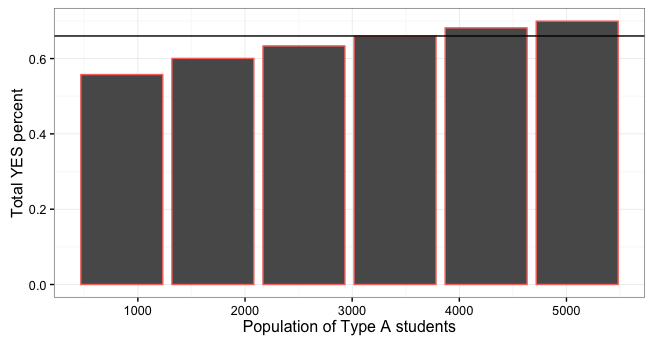It is worth noting that, in this case I fixed the ratio of hard yes voters to hard no voters at 4:1 because I didn’t think it was feasible to consider scenarios where the number of hard yes voters would exceed 10 times the number of dog owners in the town. Since there are around 300 dog owner in the town any population for Type A over 3,000 I consider pretty suspect.

## Follow up 1: The 5-Type Model

An extension of the 3-type model is a 5-type model with the following types:

1. certain yes - residents who are almost certain to vote and almost certain to vote yes
2. lean yes - voters who are likely to vote and leaning toward yes
3. toss-up - true undecideds
4. lean no - voters who are likely to vote and leaning toward no
5. certain no - voters almost certain to vote and almost certain to vote no

The simulation I will conduct with this model will proceed by making assumptions about the number of people in each group and exploring the vote probabilities and vote yes probabilities that lead to referendum wins.

I like this model because it retains a lot of the simplisity of the 3-Type Model but with a bit more added realism. It also allows us to empirically explore what I deem to be one of the more realistic real-world strategy for increasing the odds that the referendum passes: voter outreach.

The following simulation is based on a couple assumptions that are ad-hoc but not unrealistic:

1. Assume that the strongly in favor group and the strongly opposed groups are pretty calcified. Meaning that, at this point, there is not much that can be done to change the numbers or composition of either group.
2. Assume that individuals in the undecided group can be converted to ‘lean yes’ voters with some outreach.

### Simulations

The 5-Type Model proceeds in the following steps:

Step 1

Every individual in the town population is randomly assigned a type: ‘A’,’B’,’C’,’D’,’E’, corresponding to ‘hard yes’, ‘lean yes’,’indifferent’,’lean no’,’hard no.’ This is accomplished by sampling from the vector $[A,B,C,D,E]$ 17,000 times with replacement. I use sampling weights to ensure that the resulting distribution of types in the resident population follows some assumed parameters:

$p=[pA,pB,pC,pD,pE]$ are the population percentages for each type

Step 2

Once each individual has been assigned a type, that individual is then assigned a probability of voting. The probability that an individual votes is $pvote$ and is randomly generated according to:

• if individual is type A then $pvote$ is drawn from a uniform distribution with min=$pvote_{A}^{min}$ and max=$pvote_{A}^{max}$
• if individual is type B then $pvote$ is drawn from a uniform distribution with min=$pvote_{B}^{min}$ and max=$pvote_{B}^{max}$
• if individual is type C then $pvote$ is drawn from a uniform distribution with min=$pvote_{C}^{min}$ and max=$pvote_{C}^{max}$
• if individual is type D then $pvote$ is drawn from a uniform distribution with min=$pvote_{D}^{min}$ and max=$pvote_{D}^{max}$
• if individual is type E then $pvote$ is drawn from a uniform distribution with min=$pvote_{E}^{min}$ and max=$pvote_{E}^{max}$

Each individual is also randomly assigned a probability of voting yes based on that individual’s type. The probability that an individual votes ‘yes’ conditional on voting is generated according to:

• if individual is type A then $pyes$ is drawn from a uniform distribution with min=$pyes_{A}^{min}$ and max=$pyes_{A}^{max}$
• if individual is type B then $pyes$ is drawn from a uniform distribution with min=$pyes_{B}^{min}$ and max=$pyes_{B}^{max}$
• if individual is type C then $pyes$ is drawn from a uniform distribution with min=$pyes_{C}^{min}$ and max=$pyes_{C}^{max}$
• if individual is type D then $pyes$ is drawn from a uniform distribution with min=$pyes_{D}^{min}$ and max=$pyes_{D}^{max}$
• if individual is type E then $pyes$ is drawn from a uniform distribution with min=$pyes_{E}^{min}$ and max=$pyes_{E}^{max}$

Step 3

Once each individual has been assigned a type and a probability of voting, for each individual $i$ we randomly generate a $[0,1]$ value which indicates whether the individual voted or not in the current simulation. The $[0,1]$ observation is obtained by taking a single random draw from the binomial distribution with

$p(success)=pvote_{i}$

Step 4

For each individual $i$ we generate a $[0,1]$ value indicating whether the individual votes ‘yes’ or not in the current simulation. The $[0,1]$ value is again obtained by taking a single random draw from a binominal distribution with

$p(success)=pyes_{i}$

Step 5: The voter outreach step

Draw a random sample of residents with size equal to the assumed number of ‘dog-owner contacts’ (call this parameter $ncom$) by sampling the vector [1:17000] $ncom$ times without replacement. Generate a random [0,1] vector by sampling the binomial distribution with probability of success equal the conversion probability (call the conversion probability parameter $pcon$). This [0,1] vector indicates whether the resident who has been contacted by the dog owning resident changes his or her type or not. If the value equals 1, then update the resident’s type and draw a new $pvote$ and $pyes$ for that resident based on the new type.

Step 6

At this point each individual has a vote/no vote value and a yes/no value. The simulated vote for that individual is the product of these two things. At the risk of being pedantic, in order for a ‘yes’ vote the be recorded the simulated individual must have vote = 1 and vote_yes = 1.

Step 7

• Sum the total number of votes and divide by 17,000 to get the voter turnout percent
• Sum the total number of ‘yes’ votes and divide by the total number of votes to the get the ‘yes’ percent.

### Example 1: an optomistic scenario

Assumptions:

• the size of the ‘hard yes’ population is about 700 and is twice as big as the ‘hard no’ population
• assume that the ‘lean no’ group has a 50/50 chance of voting but a very low chance of voting ‘yes’ if they do vote
• assume that the ‘lean yes’ group has at least a 50% chance of voting but has a 60-80% chance of voting yes as long as they vote

Parameters:

1. $pvote \sim UNIF(0.9,1)$ and $pyes \sim UNIF(0.95,1)$ for type A
2. $pvote \sim UNIF(0.5,0.5)$ and $pyes \sim UNIF(0.6,0.8)$ for type B
3. $pvote \sim UNIF(0.2,0.3)$ and $pyes \sim UNIF(0.2,0.8)$ for type C
4. $pvote \sim UNIF(0.5,0.5)$ and $pyes \sim UNIF(0.3,0.5)$ for type D
5. $pvote \sim UNIF(0.9,1)$ and $pyes \sim UNIF(0,0.05)$ for type E
6. conversion rate = 0.5
7. number of contacts = 900
8. percent of resident population in each type is $[0.04,pB,pC,0.05,0.02]$
9. We vary $pB$ between 0.55 and 0.65 and assume that $pC=1-(pA+pB+pD+pE)$.

Preview of Results:

1. If you believe these parameters are realistic then the number of ‘lean yes’ voters required in order to win the hypothetical referendum in most cases is pretty high (greater than 65% of the town population)

2. The results are basically driven by the number of ‘lean yes’ voters you assume there are in the population.

A few points to consider:

1. Although the number of ‘lean yes’ voters is pretty high (11,000 out of 17,000 residents) the threshold for being a ‘lean yes’ voter is not all that high: ‘lean yes’ still only means 50/50 odds of showing up to vote and a 60-80% of voting yes conditional on showing up.

#---------------------------------------------
# a hypothetical 'get out the vote' campaign.. this is a little complicated but
# we are going to do the following:

#1. assume every resident has a type but we cannot observe the type
#2. assume that each interaction between a dog owner and a
#   non-dog owner has a chance of changing the non-dog owner's type as long as the
#   non-dog owner does not belong to the 'no' group.
# 2A. assume that only dog owners and a few close associates of dog owners are in the 'yes' group.
# 2B. assume the probabilities of a type change are as follows:
#    --- if the non-dog owner is in the 'lean yes' group, the interaction has a 50% chance
#          of increasing that resident's probability of voting and has a 20% chance of
#          increasing that residents's probability of voting yes.
#   --- if the non-dog owner is in the 'indifferent' group then the interaction has a
#          20% chance of moving the resident to the 'lean yes' group
#   --- if the non-dog owner is in the 'lean no' group then the interaction has a 20% #   #			chance
#          of moving them to the 'indifferent' group
#   --- if the non-dog owner is in the 'no' group there is no possibility of changing the #			resident's type

outreach.fn <- function(ncom,conversion.rate,pA,pB,pD,pE){
pA <- pA
pB <- pB
pD <- pD
#  pD <- 0.5*pB
pE <- pE
pC <- 1-(pA+pB+pD+pE)
types <- sample(c("A","B","C","D","E"),17000,prob=c(pA,pB,pC,pD,pE),replace=TRUE)

pop <- data.frame(type=types)

pop$pvote <- 0 pop$pvote[which(pop$type=='A')] <- runif(length(pop$type[pop$type=='A']),0.9,1) pop$pvote[which(pop$type=='B')] <- runif(length(pop$type[pop$type=='B']),0.5,0.5) pop$pvote[which(pop$type=='C')] <- runif(length(pop$type[pop$type=='C']),0.2,0.2) pop$pvote[which(pop$type=='D')] <- runif(length(pop$type[pop$type=='D']),0.5,0.5) pop$pvote[which(pop$type=='E')] <- runif(length(pop$type[pop$type=='E']),0.9,1) pop$pyes <- 0
pop$pyes[which(pop$type=='A')] <- runif(length(pop$type[pop$type=='A']),0.95,1)
pop$pyes[which(pop$type=='B')] <- runif(length(pop$type[pop$type=='B']),0.6,0.8)
pop$pyes[which(pop$type=='C')] <- runif(length(pop$type[pop$type=='C']),0.2,0.8)
pop$pyes[which(pop$type=='D')] <- runif(length(pop$type[pop$type=='D']),0.3,0.5)
pop$pyes[which(pop$type=='E')] <- runif(length(pop$type[pop$type=='E']),0.0,0.1)

pop$pvote_new <- pop$pvote
pop$pyes_new <- pop$pyes
pop$type_new <- pop$type
#now sample the row numbers of this data frame 100 times
idx.change <- sample(c(1:17000),ncom,replace=FALSE)
p.change <- rbinom(ncom,1,conversion.rate)

k<-1
for(i in idx.change){

t <- pop$type[i] if(p.change[k]==1){ if(t=='B'){ pop$pvote_new[i]<-pop$pvote[i]+0.1 pop$pyes_new[i] <- pop$pyes[i] + 0.05 } if(t=='C'){ pop$type_new[i] <- 'B'
pop$pvote_new[i] <- runif(1,0.5,0.8) pop$pyes_new[i] <-   runif(1,0.5,0.8)
}

if(t=='D'){
pop$type_new[i] <- 'C' pop$pvote_new[i] <- runif(1,0.2,0.6)
pop$pyes_new[i] <- runif(1,0.2,0.6) } } k<-k+1 } #add a column simulating vote/not vote pop$vote=unlist(lapply(pop$pvote_new,rbinom,n=1,size=1)) pop$vote_yes=unlist(lapply(pop$pyes_new,rbinom,n=1,size=1)) #tally the yes votes pop$Yes <- pop$vote*pop$vote_yes

#score the vote
turnout <- sum(pop$vote)/17000 totalyes <- sum(pop$Yes)/sum(pop$vote) return(data.frame(ncom=ncom,turnout=turnout,totalyes=totalyes, nC=nrow(pop[which(pop$type_new=='C'),]),pB=pB,conversion.rate))
}
#--------------------------------------------
#####################################################################################
#####################################################################################
#####################################################################################
#####################################################################################
#####################################################################################
#####################################################################################
#####################################################################################
#####################################################################################
#####################################################################################
#####################################################################################
#####################################################################################

#================================================================
#An Optomistic Scenario

# assume that the A group is twice as large as the E group
# assume that the 'lean no' group has a 50/50 chance of voting
# assume that the 'lean yes' group has at least a 50% chance or better
#    voting and has a 60-80% chance of voting yes as long as they vote

scen1 <- data.frame(rbindlist(lapply(c(rep(0.5,50),rep(0.55,50),rep(0.6,50),rep(0.65,50)),
outreach.fn,
conversion.rate=0.5,ncom=900,pA=0.04,pD=0.05,pE=0.02)))
ggplot(scen1,aes(x=pB,y=totalyes,group=pB,color='red')) + geom_boxplot()  +
guides(colour=FALSE) + xlab("Type B population percent") + ylab('Percent voting YES')

#================================================================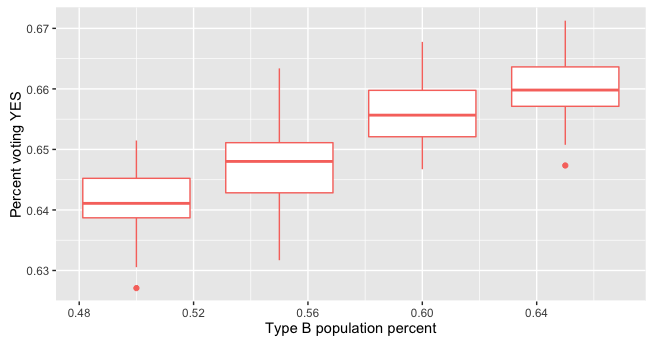From these results the core conclusions remain pretty unchanged:

• if the hard yes group is about twice as big as the hard no group
• and if the hard yes group is about 600-700 residents
• and if the lean yes group has a 50% chance of showing up and a 60-80% of voting yes if they do show up

then we need around 60% of the town in the lean yes category.

### Example 2: Fewer hard no residents

When I ran my ad-hoc parameters by some people with intimate knowledge of the totally fake world I’ve made up here, an overwhelming sentiment emerged that the hard no group is probably smaller than I’m imagining. Most people I talked to seem to think that the hard yes group is more like 4 times as large as the hard no group…rather than 2 times as large.

Though this might be overly optomistic, I’ll run it just for illustrative purposes.

scen1 <- data.frame(rbindlist(lapply(c(rep(0.5,50),rep(0.55,50),rep(0.6,50),rep(0.65,50)),outreach.fn,
conversion.rate=0.5,ncom=900,pA=0.04,pD=0.05,pE=0.01)))
ggplot(scen1,aes(x=pB,y=totalyes,group=pB,color='red')) + geom_boxplot()  +
guides(colour=FALSE) + xlab("Type B population percent") + ylab('Percent voting YES')



Here, I’ve kept the size of the hard yes group the same at 680 residents but I’ve decreased the size of the hard no group. The assumption in this model is that lean no population from the last simulation stays the same (meaning that all the simulated residents that I took out of the hard no group went into the toss-up group).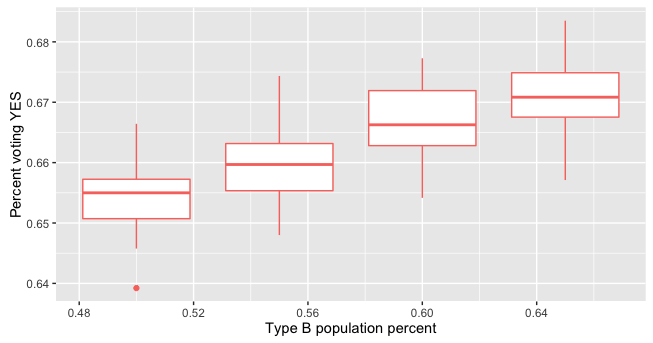If my expert opinions’ optimism is warranted and the hard no group really is smaller than I think, then the dog park could conceivably pass with as few as 55% of the residents in the lean yes group.

## Final simulation: the lobbying model

Here I vary two parameters simultaneously:

1. $ncom$ the number of contacts that each dog-owner makes while lobbying for the dog park
2. the conversion rate. i.e. how many residents out of every 10 contacts is swayed to join the ‘lean yes’ group.

The interesting thing to note here is that there are two source of uncertainty regarding how many “indifferent” or “lean no” residents can be converted to “lean yes” residents:

1. the dog-owner cannot observe a persons type before the contact. This means that, when the contact is initiated, there is: i) some probability that the dog-owner will be engaging somebody who is already in the “lean yes” category (in which case the contacted individuals probability of voting in favor of the dog park increases only slightly, and ii) some probability that the dog-owner is engaging somebody from the “hard no” group who has no chance of being converted.

2. Even if the contact that is initiated is with an “indifferent” resident, it is still not certain that the contacted resident will join the “lean yes” group. The parameter $conversion.rate$ determines how likely it is that a contact with a non-dog owner will result in a conversion to the “lean yes” group.

The key question here is:

• if the “hard yes” group is 4% of the town population, and
• the “hard no” group is 2% of the town population, and
• the initial “lean yes” group is 40% of the town population, and
• the initial “lean no” group is 5% of the town population

how many total contacts do the dog-owners need to make and what conversion rates do they need in order to get the dog park passed?

outreach.fn <- function(ncom,conversion.rate,pA,pB,pD,pE){
pA <- pA
pB <- pB
pD <- pD
#  pD <- 0.5*pB
pE <- pE
pC <- 1-(pA+pB+pD+pE)
types <- sample(c("A","B","C","D","E"),17000,prob=c(pA,pB,pC,pD,pE),replace=TRUE)

pop <- data.frame(type=types)

pop$pvote <- 0 pop$pvote[which(pop$type=='A')] <- runif(length(pop$type[pop$type=='A']),0.9,1) pop$pvote[which(pop$type=='B')] <- runif(length(pop$type[pop$type=='B']),0.6,0.8) pop$pvote[which(pop$type=='C')] <- runif(length(pop$type[pop$type=='C']),0.2,0.2) pop$pvote[which(pop$type=='D')] <- runif(length(pop$type[pop$type=='D']),0.6,0.6) pop$pvote[which(pop$type=='E')] <- runif(length(pop$type[pop$type=='E']),0.9,1) pop$pyes <- 0
pop$pyes[which(pop$type=='A')] <- runif(length(pop$type[pop$type=='A']),0.95,1)
pop$pyes[which(pop$type=='B')] <- runif(length(pop$type[pop$type=='B']),0.6,0.8)
pop$pyes[which(pop$type=='C')] <- runif(length(pop$type[pop$type=='C']),0.2,0.8)
pop$pyes[which(pop$type=='D')] <- runif(length(pop$type[pop$type=='D']),0.3,0.5)
pop$pyes[which(pop$type=='E')] <- runif(length(pop$type[pop$type=='E']),0.0,0.1)

pop$pvote_new <- pop$pvote
pop$pyes_new <- pop$pyes
pop$type_new <- pop$type
#now sample the row numbers of this data frame 100 times
idx.change <- sample(c(1:17000),ncom,replace=FALSE)
p.change <- rbinom(ncom,1,conversion.rate)

k<-1
for(i in idx.change){

t <- pop$type[i] if(p.change[k]==1){ if(t=='B'){ pop$pvote_new[i]<-pop$pvote[i]+0.1 pop$pyes_new[i] <- pop$pyes[i] + 0.05 } if(t=='C'){ pop$type_new[i] <- 'B'
pop$pvote_new[i] <- runif(1,0.5,0.8) pop$pyes_new[i] <-   runif(1,0.5,0.8)
}

if(t=='D'){
pop$type_new[i] <- 'C' pop$pvote_new[i] <- runif(1,0.2,0.6)
pop$pyes_new[i] <- runif(1,0.2,0.6) } } k<-k+1 } #add a column simulating vote/not vote pop$vote=unlist(lapply(pop$pvote_new,rbinom,n=1,size=1)) pop$vote_yes=unlist(lapply(pop$pyes_new,rbinom,n=1,size=1)) #tally the yes votes pop$Yes <- pop$vote*pop$vote_yes

#score the vote
turnout <- sum(pop$vote)/17000 totalyes <- sum(pop$Yes)/sum(pop$vote) return(data.frame(ncom=ncom,turnout=turnout,totalyes=totalyes, nC=nrow(pop[which(pop$type_new=='C'),]),pB=pB,conversion.rate))
}
#--------------------------------------------

#fix everything else and vary the conversion rate
scen1 <- data.frame(rbindlist(lapply(c(rep(0.5,50),rep(0.6,50),rep(0.7,50),rep(0.8,50)),outreach.fn,
ncom=6000,pA=0.04,pB=0.4,pD=0.05,pE=0.02)))
ggplot(scen1,aes(x=conversion.rate,y=totalyes,group=conversion.rate,color='red')) + geom_boxplot()  +
guides(colour=FALSE) + xlab("Conversion Rate") + ylab('Percent voting YES')

#now vary the conversion rate and the total outreach number
com1 <- data.frame(rbindlist(lapply(c(rep(0.5,50),rep(0.6,50),rep(0.7,50),rep(0.8,50)),outreach.fn,
ncom=6000,pA=0.04,pB=0.4,pD=0.05,pE=0.02)))

com2 <- data.frame(rbindlist(lapply(c(rep(0.5,50),rep(0.6,50),rep(0.7,50),rep(0.8,50)),outreach.fn,
ncom=8000,pA=0.04,pB=0.4,pD=0.05,pE=0.02)))

com3 <- data.frame(rbindlist(lapply(c(rep(0.5,50),rep(0.6,50),rep(0.7,50),rep(0.8,50)),outreach.fn,
ncom=10000,pA=0.04,pB=0.4,pD=0.05,pE=0.02)))

z <- rbind(com1,com2,com3)

ggplot(z,aes(x=factor(ncom),y=totalyes,fill=factor(conversion.rate))) + geom_boxplot() +
scale_fill_brewer(palette='blues') +
theme_bw() +
xlab("number of contacts") +
ylab("percent voting yes")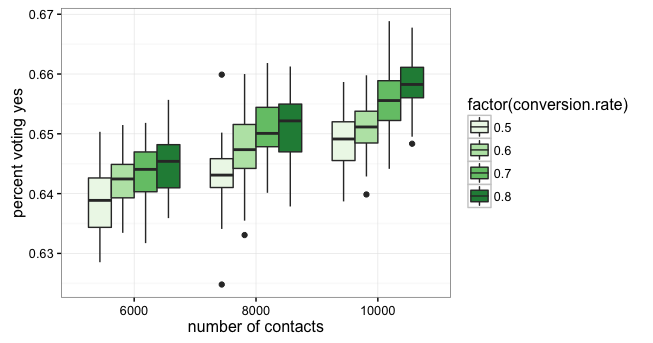In this case, if each dog owner only contacts about 20 people there is a very low probability of the dog park ballet measure passing (even with an 80% conversion rate for non-dog owning contacts. Interestingly, even in the neighborhood of 30 contacts per dog-owner, there exists decent chances for success only at very high conversion rates.

One thing I would take from this if I were a dog owner is the importance of contacting as many town residents as possible in order to get as many people as possible into the “lean yes” group. Think about it this way:

if all the dog owners got together and formed an agreement that they would each get 20 town residents into the “lean yes” category, how many people would each resident have to talk to in order to get those 20 “lean yes” residents?

This, again, is simple to express analytically but still informative:

• according to the lobbying model a resident can only be converted to “lean yes” if they are in the “indifferent” category. Recall that “lean no” voters can be converted to “indifferent” and “hard yes” and “hard no” can’t be changed.
• The starting parameters dictate that 4% of the town population is “hard yes”, 40% is “lean yes”, 5% is “lean no”, and 2% is “hard no.”
• This leaves 49% of the population in the “indifferent” category.

For any contact between dog-owner $i$ and town resident $j$, the odds of $i$ converting $j$ to the “lean yes” category is:

For illustrative purposes, peg the conversion probability at something very high like 80%, then

which is

We can again leverage the binomial distribution. Consider the event:

dog owner $i$ converts town resident $j$ to “lean yes”

to be a success. How many trials, on average, need to be performed in order to get 20 successes if the probability of a success on any one trial is 0.392? The expected number of successes in $n$ trials with success probability of $p$ is

If we want $E(count)=20$ then we need:

or

or

Translating this into aggregate terms, this would be consistent with a value of 15,000 for the parameter $ncom$ in our model (300 dog-owners each contacting 50 people, or approximately 15,000 total resident contacts)

### How many converts do we need

In the example above, I showed that if the goal is to get 20 additional “lean yes” town residents (meaning in addition to the 40% of the town population that starts in the “lean yes” category) per dog owner, that each dog owner would have to contact around 50 people (assuming a conversion rate of 0.8). A follow up question here:

how many “lean yes” converts do we need in order to pass the ballot measure? The answer here is embedded in what I have presented above, I just want to take a second to put it into sharper focus.

We can again do this analytically…but it’s way more fun to do it numerically (that’s what computer are for right?)

The plot below shows the final vote tally as a function of the number of town residents converted to the “lean yes” group. We also normalize this population by the number of dog owners to show the number of contacts per dog owner that are consistent with the dog park ballot measure passing, conditional on assumed values for all other parameters…just to be concrete:

• the iniitial population is 4% “hard yes”, 40% “lean yes”, 49% “indifferent”, 5% “lean no”, and 2% “hard no”
• the conversion rate for dog-owner/townsperson contacts is set at 75%
• the “lean yes” group has between 60-80% change of voting and a 60-80% chance of voting yes
• the indifferent group has a 20% of voting and anywhere from a 20-80% of voting yes if they vote
• the “lean no” group has a 60-80% change of voting and a 60-80% chance of voting no if they vote
# how many 'lean yes' people do we need in the final population to start getting a win
com <- runif(1000,6000,12000)
com5 <- data.frame(rbindlist(lapply(com,outreach.fn,
conversion.rate=0.75,pA=0.04,pB=0.4,pD=0.05,pE=0.02)))
com5$convert.per.owner <- (com5$nB-6800)/300
ggplot(com5,aes(x=convert.per.owner,y=totalyes)) + geom_point(shape=1) + geom_hline(yintercept=0.66) +
geom_smooth(method="lm") + theme_bw()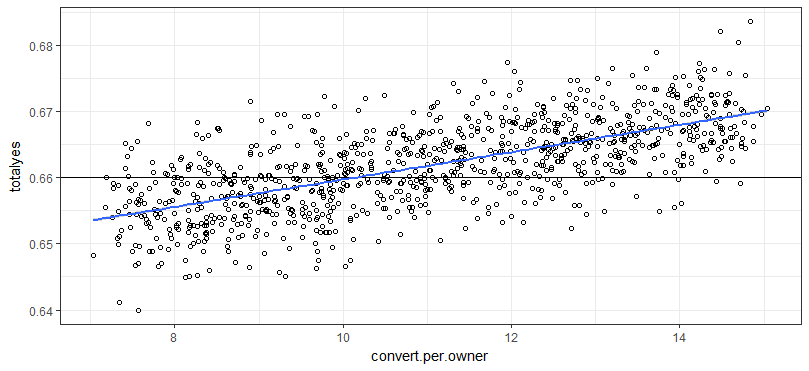The thing to note from the plot above is that, althought each dog-owner would have to reach out to about 50 people in order to get 20 converted “lean yes” voters per dog-owner, the dog-owners don’t actually need 20 converts each in order to get the dog park. With the assumed type-specific probabilities I have specified here, the dog-owners really only need about 12 to 13 converts each in order to get simulated election results where the dog park passes 70 - 80% of the time. And by the time we get to 14 converts per dog-owner, simulated election results come out in favor of the dog park pretty much every time.

The last thing to say on this point is: with 12-14 converts to the “lean yes” category per dog-owner, the final “lean yes” population is about 60% of the total town population. This emphasizes kind of the ‘no-doh’ nature of this entire post. Basically, if you need to pass a ballot measure by getting $\frac{2}{3}$ of the voters to vote “yes” then you need about $\frac{2}{3}$ of the population to be generally in favor of the measure. This is trivial and obvious but I thought it was kind of fun to think about some of the different ways you could end up getting around $\frac{2}{3}$ of voters to vote in favor of a dog park.

Written on May 16, 2017